## Actinides and the $r$-processLA-UR-19-30941

### Matthew Mumpower

Workshop on nuclear physics for astrophysical phenomena

Wednesday Oct. 23$^{rd}$ 2019Center for Theoretical
ASTROPHYSICS

## To understand the formation of the elements

Requires deep knowledge of a range of fields, including:

The theoretical modeling of astrophysical environments

Multi-messenger observations (gravitational waves, EM waves, etc.)

Nuclear theory predictions for exotic nuclei

Precision experiments to constrain nuclear theory

Data and observations are limited

We must be clever when deciphering what is going on with nucleosynthesis...## When we model nucleosynthesis

We want to describe the abundances observed in nature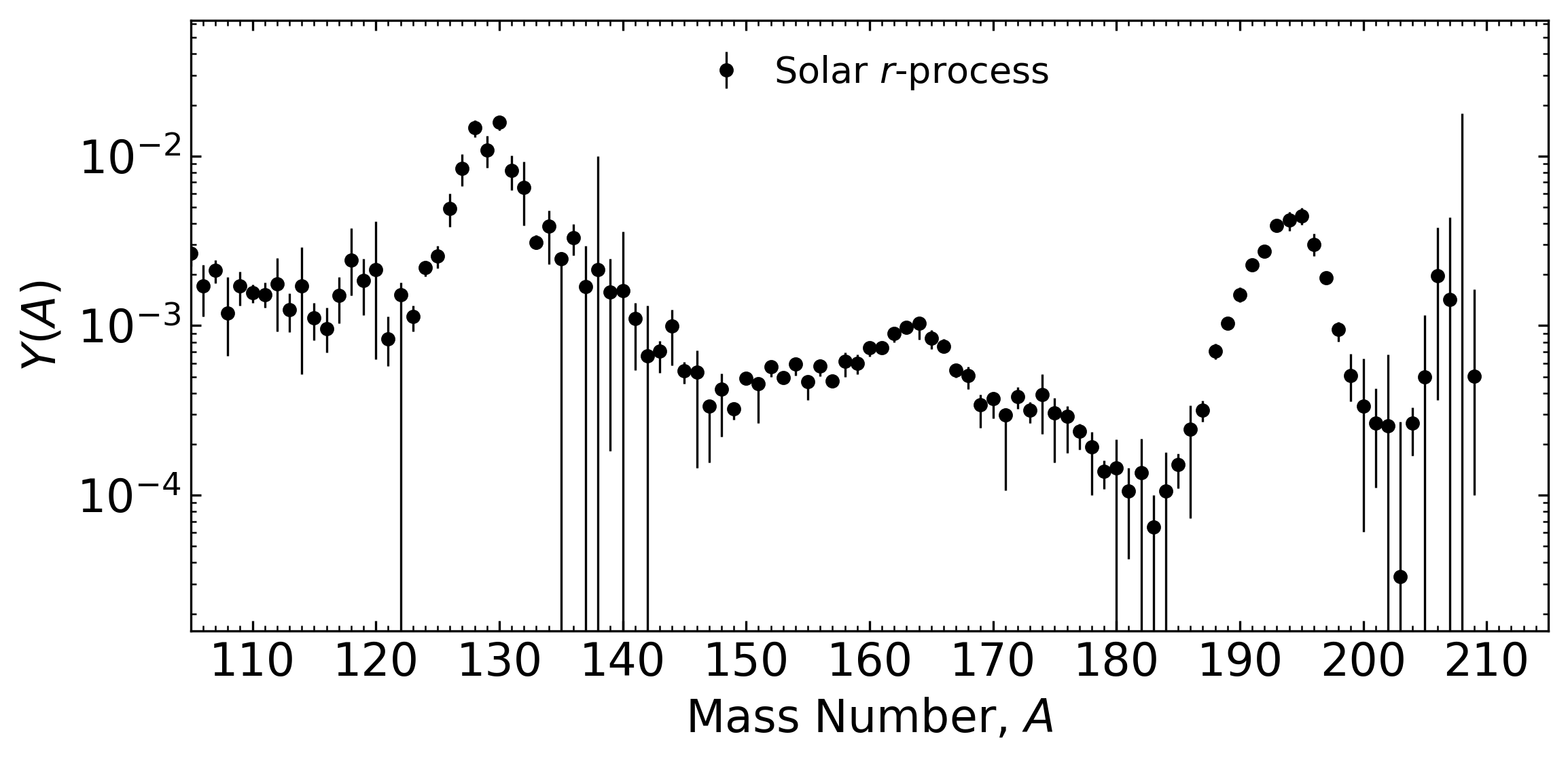But there is uncertainty in:

The astrophysical conditions (large variations in current simulations)

The nuclear physics inputs (1000's of unknown species / properties)

Arnould et al. Phys. Rep. (2007) • Metzger et al. MNRAS (2010) • Mumpower et al. PRC (2015) • Côté et al. ApJ (2018) • Cowan et al. (2019)

## Inputs from nuclear physics

1st order: masses, $\beta$-decay rates, reaction rates & branching ratios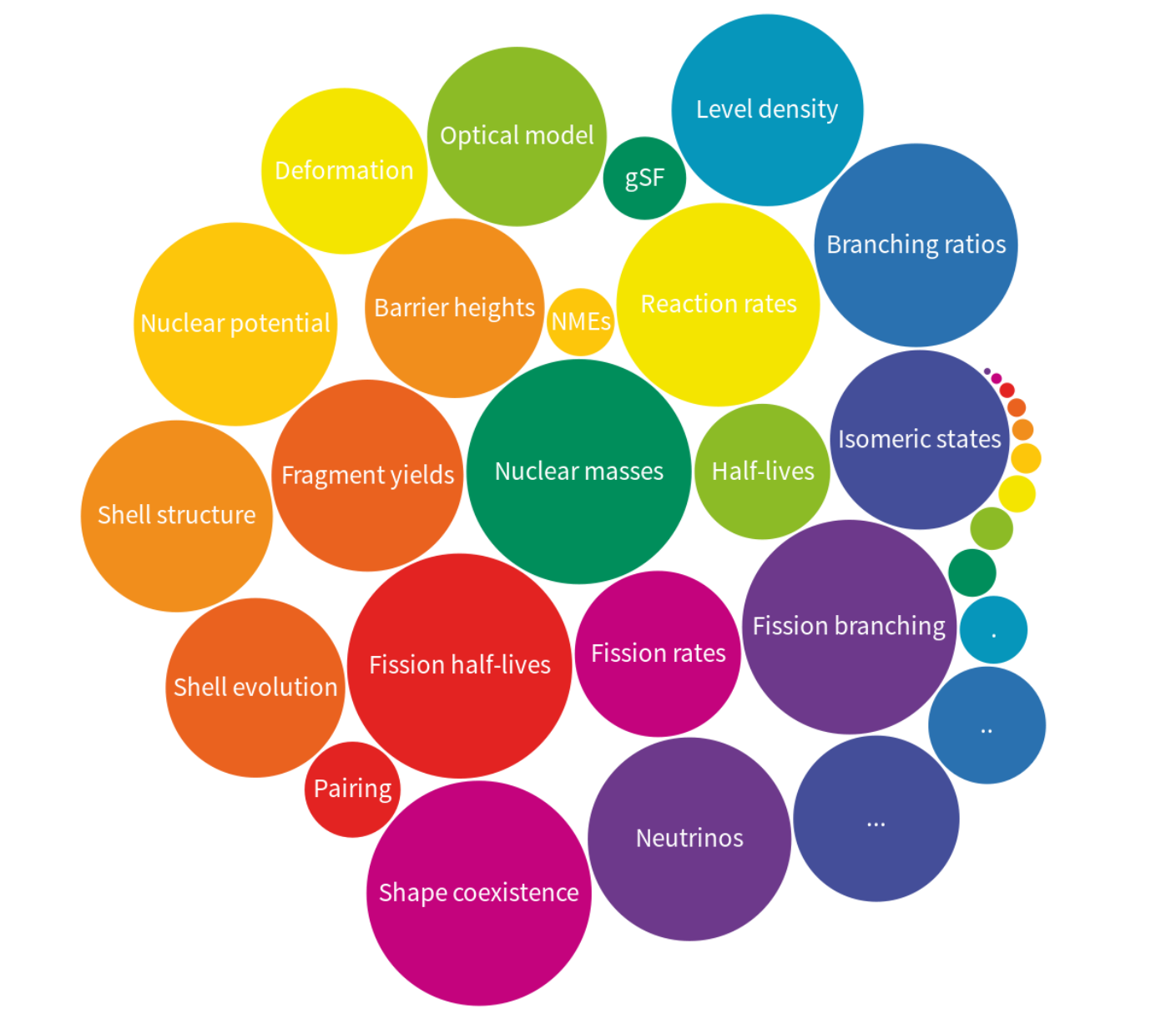Dillmann et al. EPJA (2002) • Aprahamian et al. (2018) • See review paper: Mumpower et al. PPNP 86 (2016)

## What do we know?

The chart of nuclides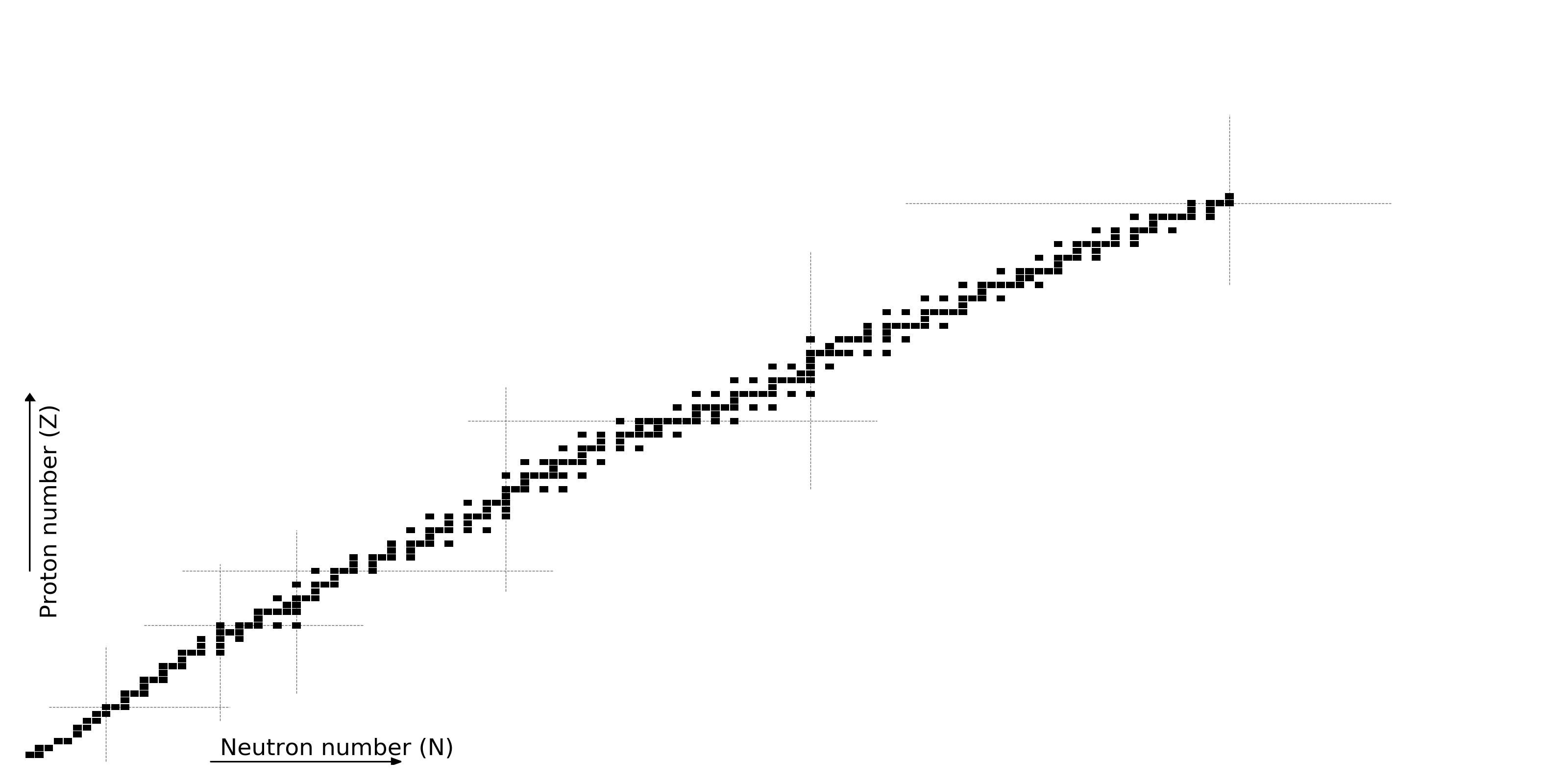Figure by Mumpower

## What do we know?

All half-lives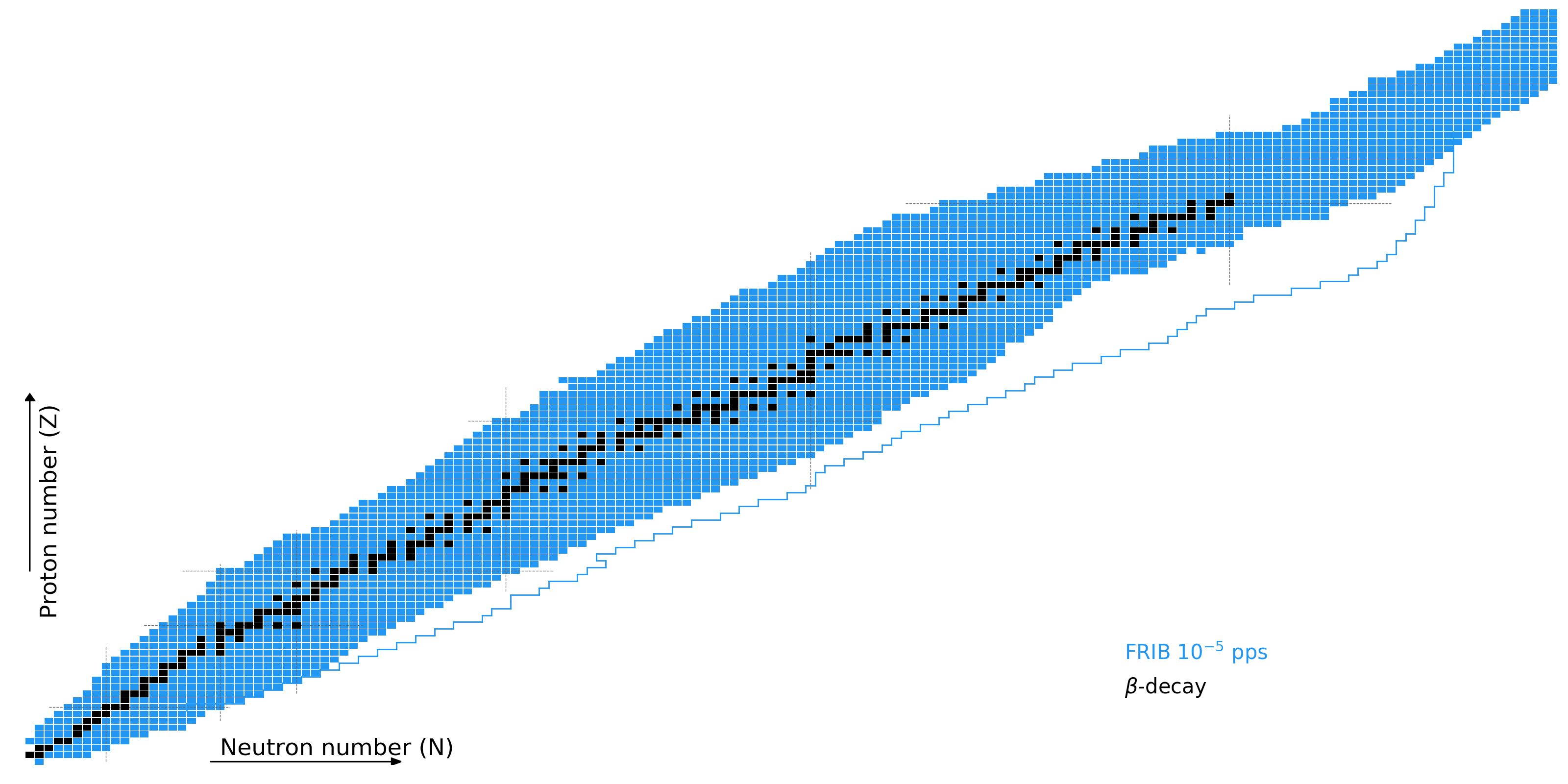Figure by Mumpower

## What do we know?

Nuclear masses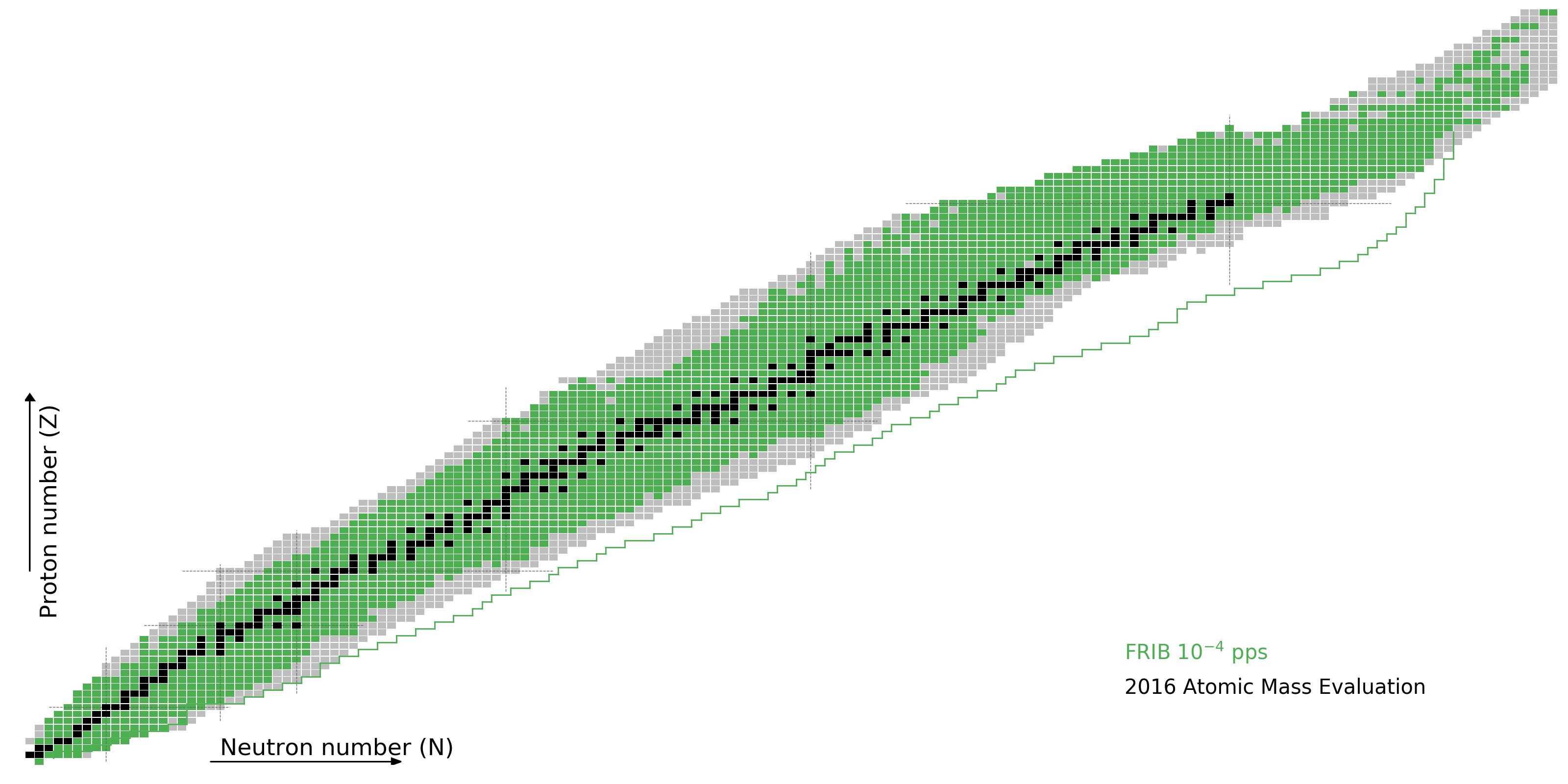Figure by Mumpower

## What do we know?

Neutron capture ratesFigure by Mumpower

## What do we know?

As of today, to varying degrees of accuracy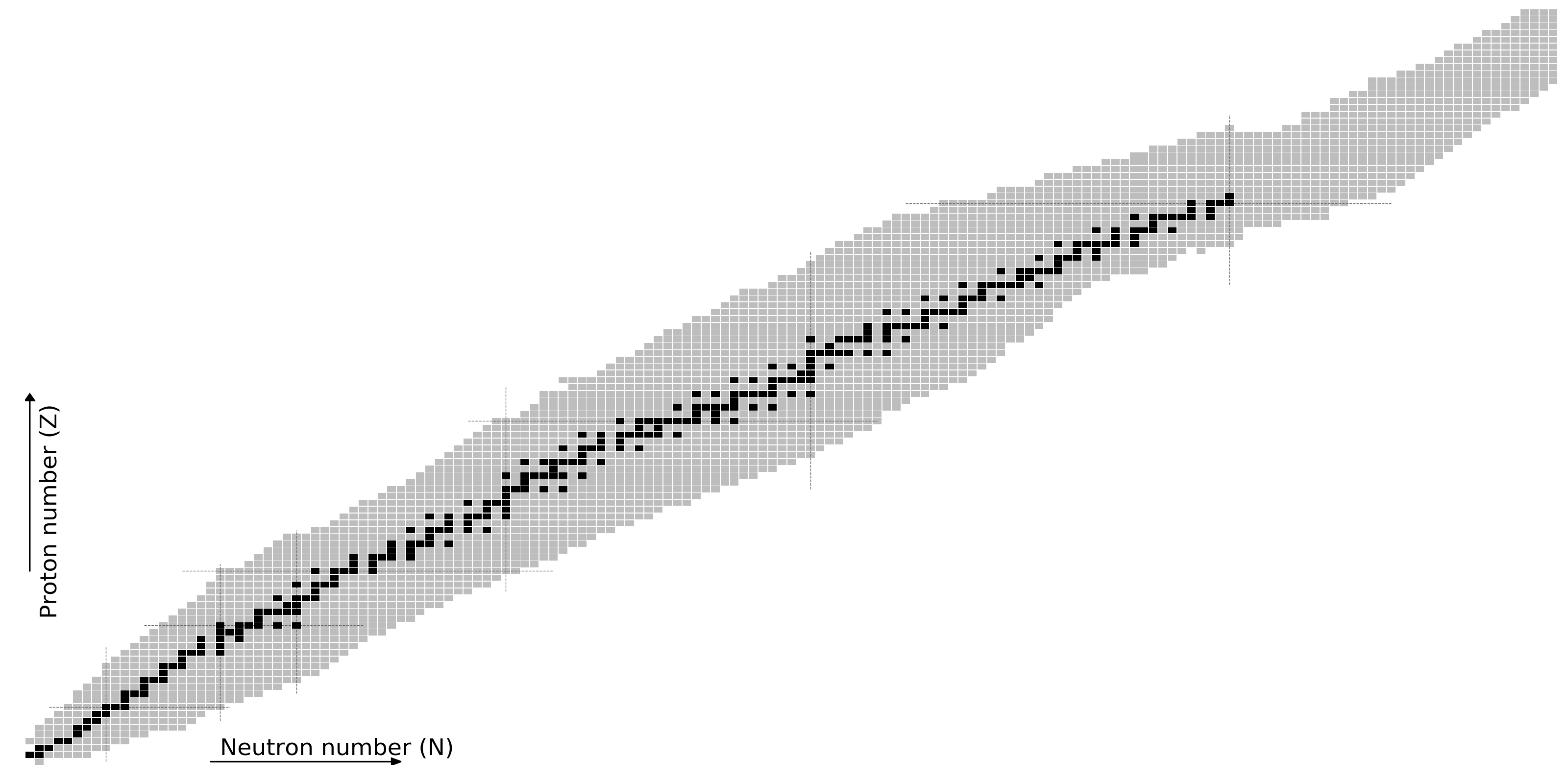Figure by Mumpower

## The r-process requires nuclear theory

Even in the era of FRIB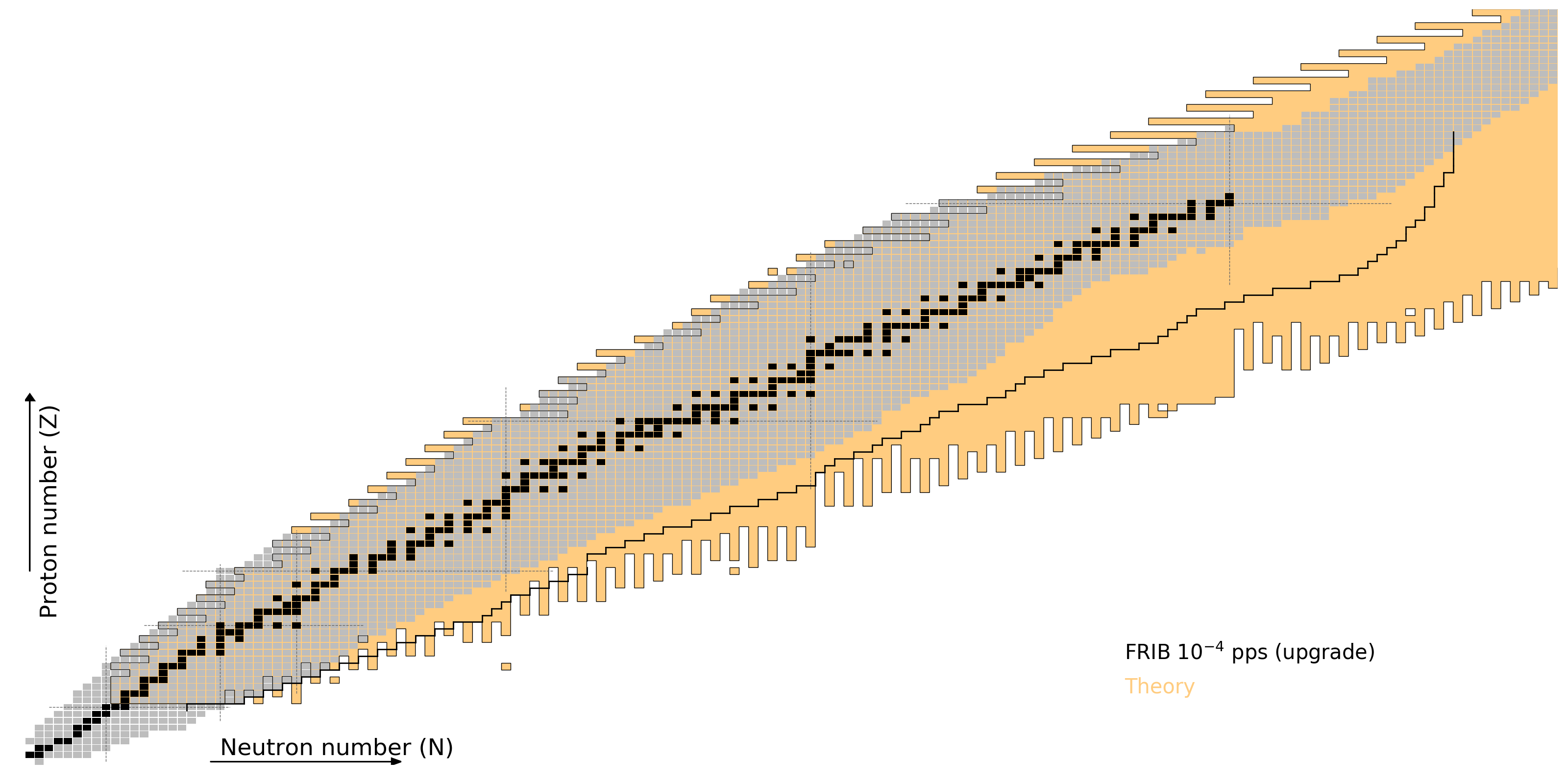Figure by Mumpower

## $r$-Process CalculationPRISM: Portable Routines for Integrated nucleoSynthesis Modeling

Sprouse & Mumpower in prep (2019)

## Distribution of $Y_e$ for merger disk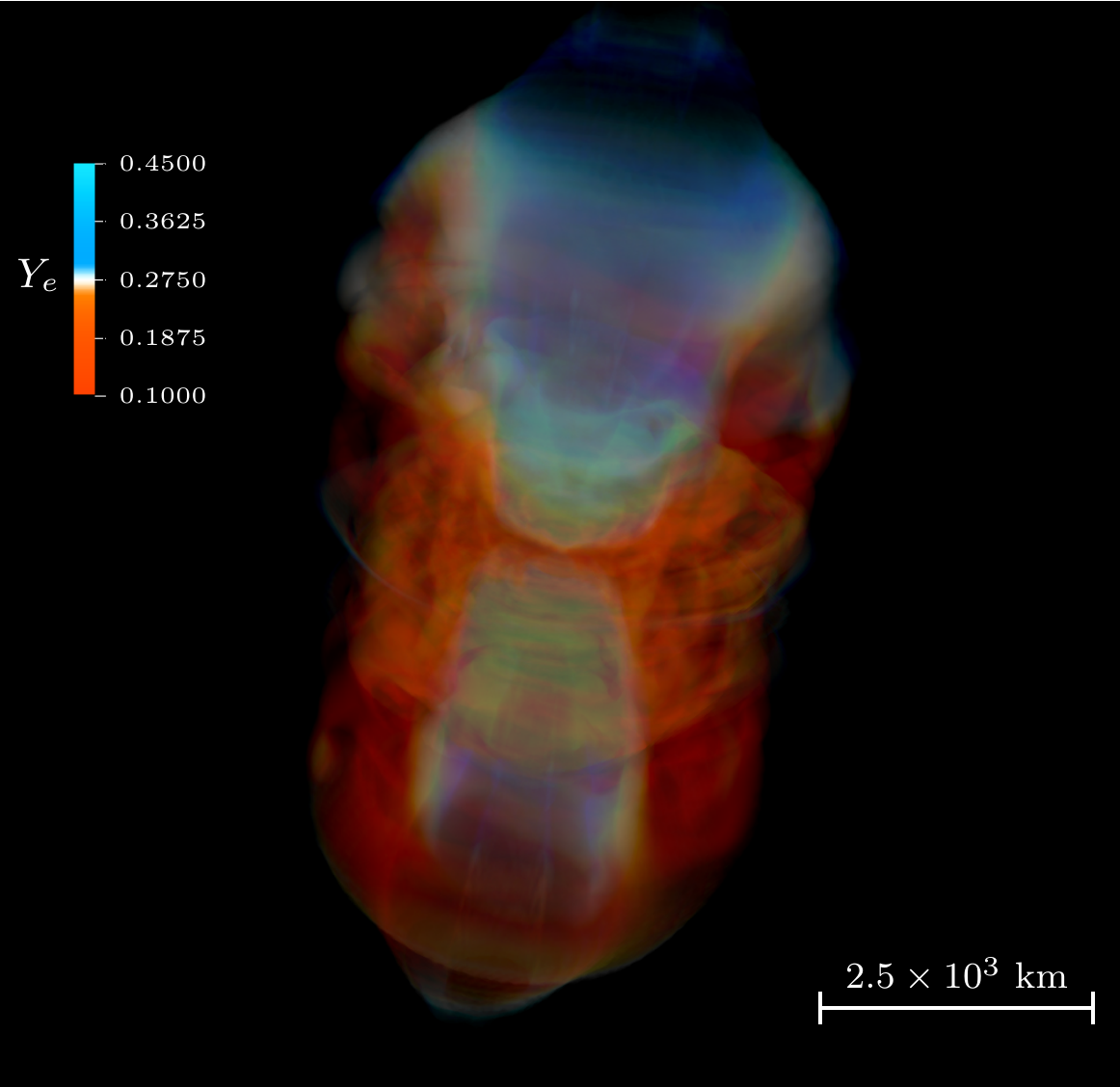Volume rendering of electron fraction, $Y_e$ for a neutron star merger disk

Jet drives material and voids region near azimuthal axis; high $Y_e$

Miller et al. PRD 100 023008 (2019)

## Morphology of ejecta

Miller et al. PRD 100 023008 (2019)

## Accretion Disk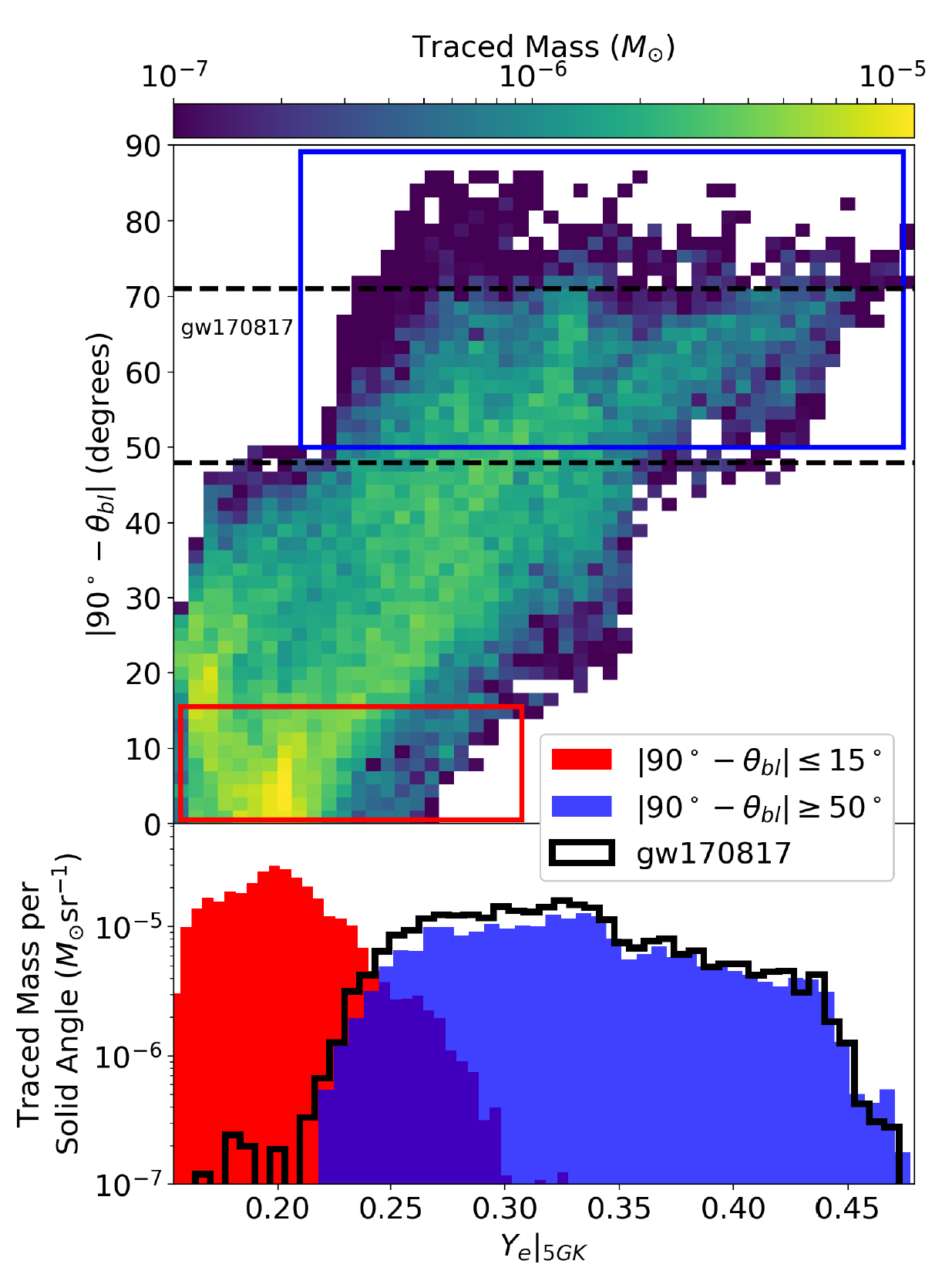Surrounding a black hole

Viewing angle critical for what composition you see

Measured from the mid-plane of the disk

Our model is consistent with observations of a blue kilonova

Red, full $r$-process material seen near mid-plane

The disk can contain a large amount of material

But exactly how much is gravitationally unbound?

Further... What is the important role of neutrinos?

Miller et al. PRD 100 023008 (2019)

## Neutrinos impact the nucleosynthesis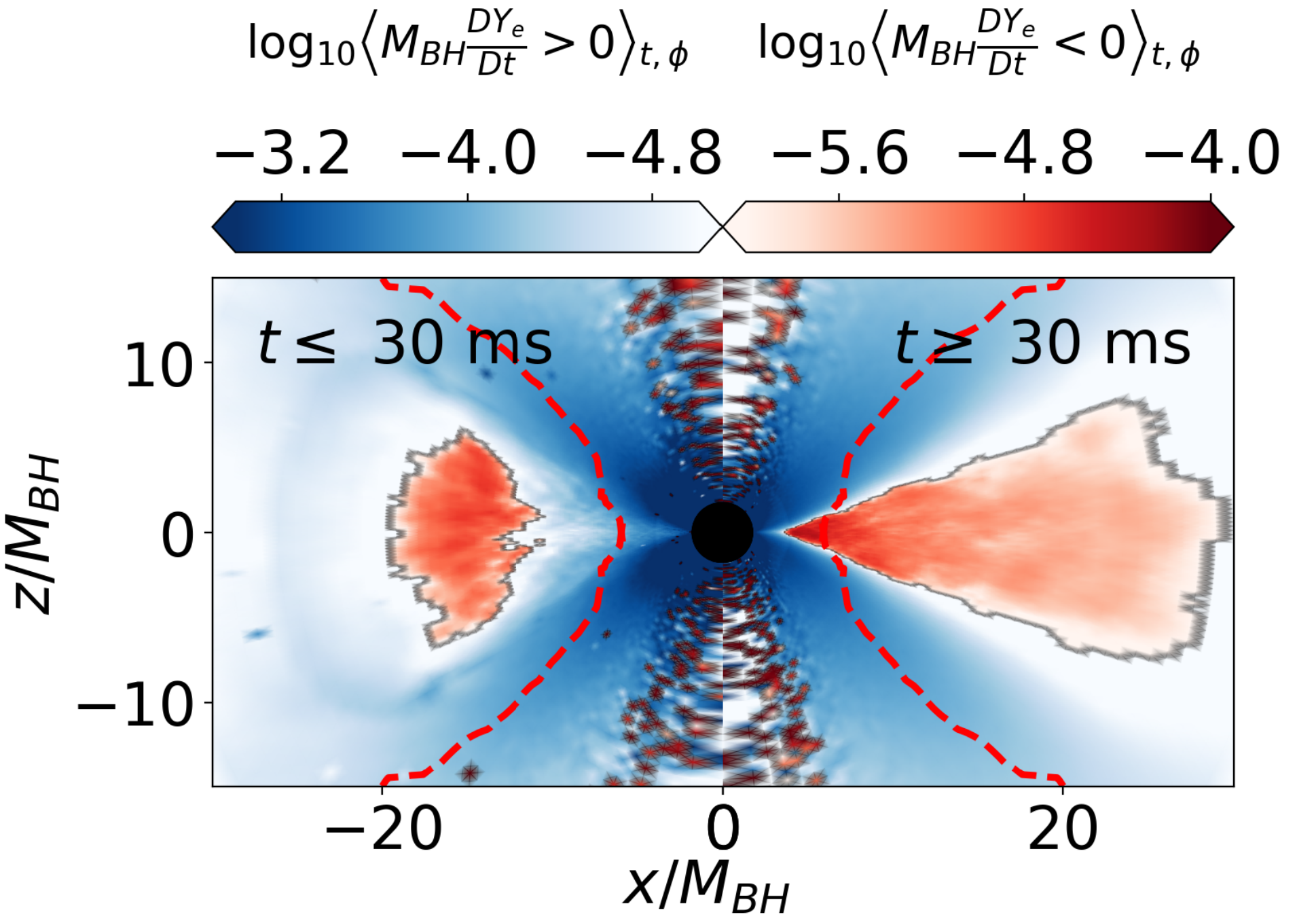Open question: exactly how is the ejecta processed by neutrinos?

Blue region has high $Y_e$; red lower $Y_e$

The transit time away from the disk is crucial

Miller et al. PRD 100 023008 (2019)

## Resultant nucleosynthesis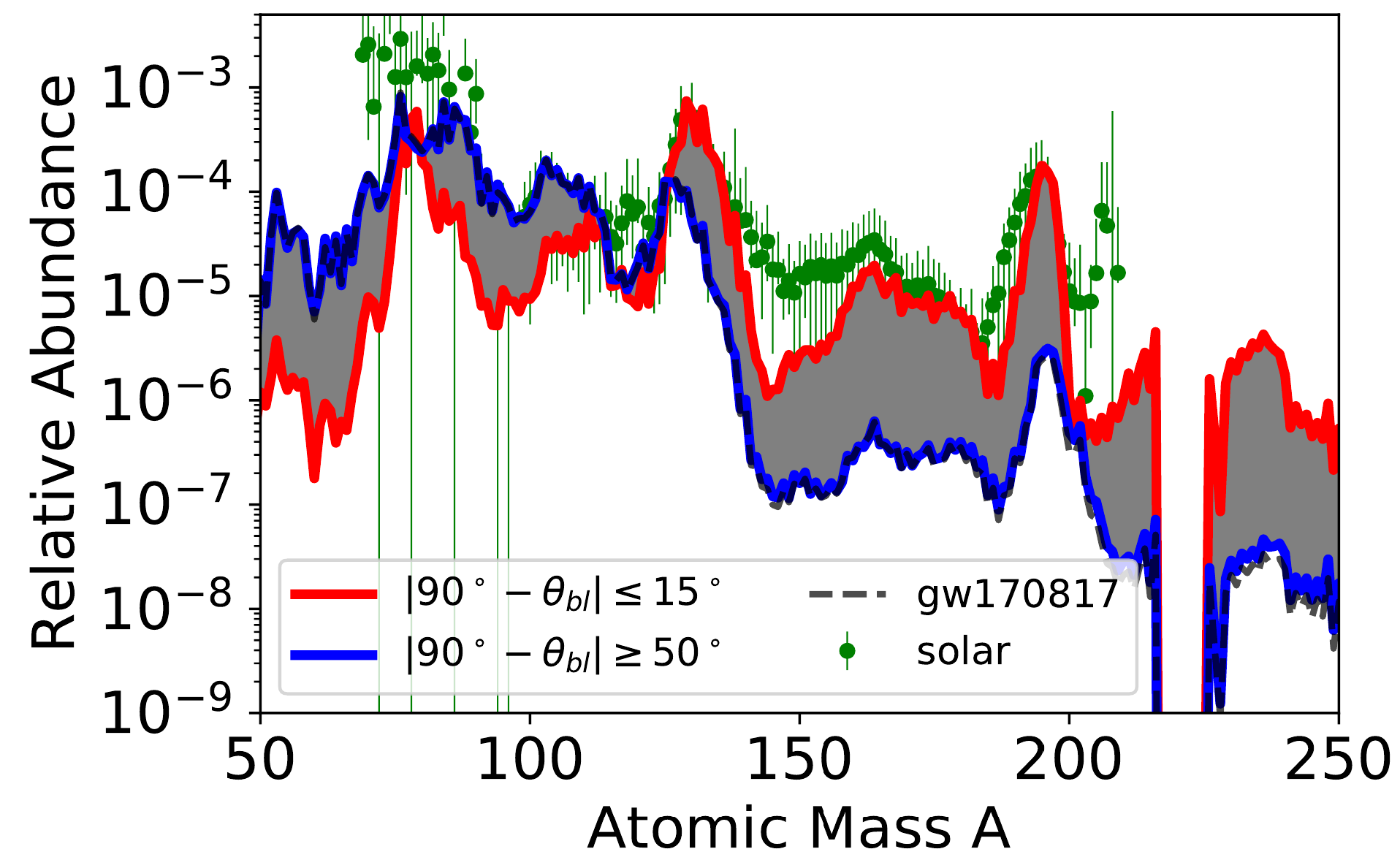Nucleosynthesis simulation with J. Lippuner's SkyNet

Mass weighted trajectories suggest blue kilonova if viewing angle is similar to GW170817

It is critical to get the full morphology and thereby composition correct...

Miller et al. PRD 100 023008 (2019)

## Inferring composition is tricky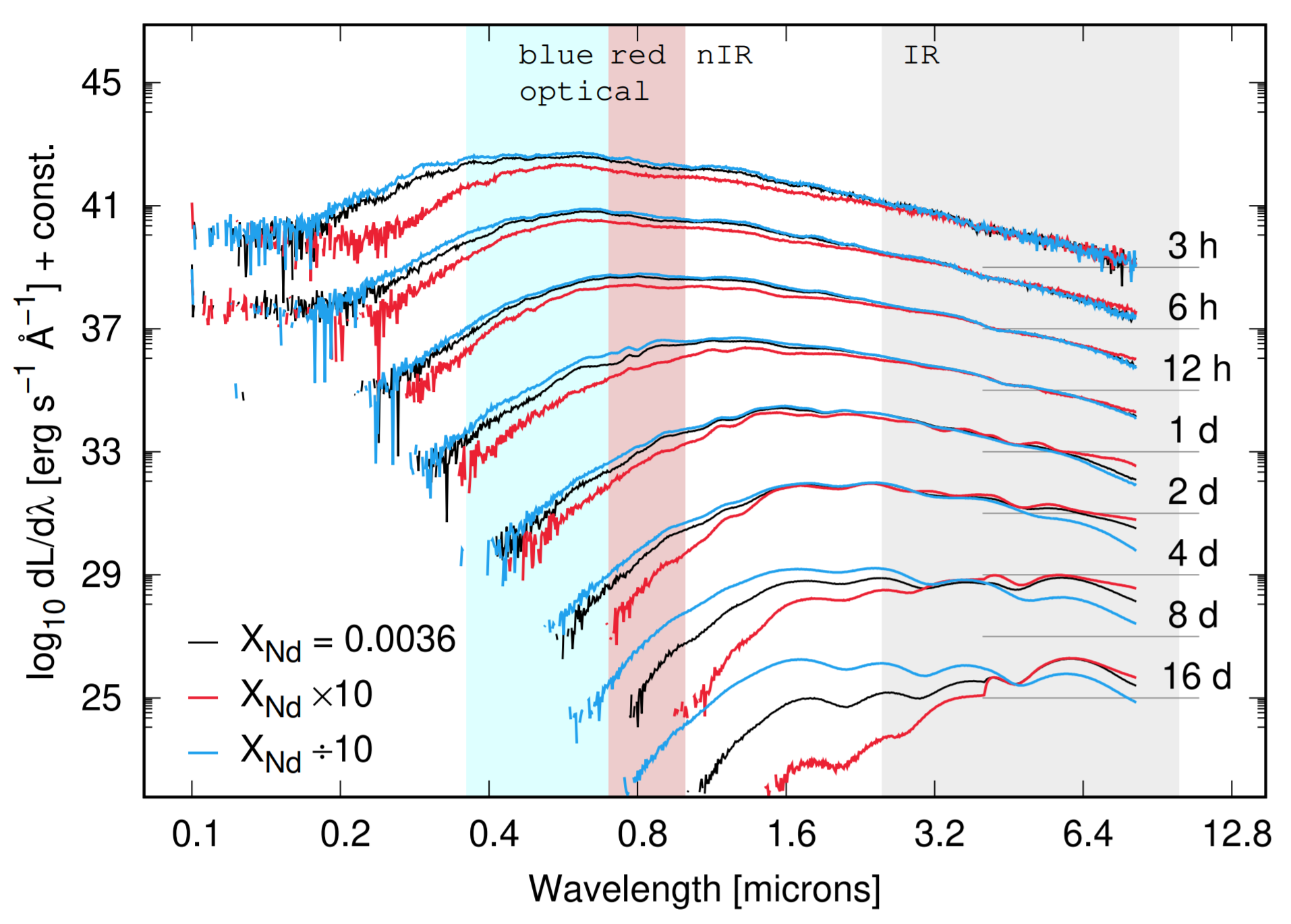One way is to find a proxy in the observed light curve spectrum

Nd is a good choice because it is a high opacity lanthanide

The amount of Nd is absolutely crucial to the observed light curve

But it is important to remember that there are still many astrophysical model degeneracies as this point...

Kasen et al. Nature (2017) • Côté et al. ApJ (2018) • Even et al. submitted (2019)

## Especially for solar-like ratios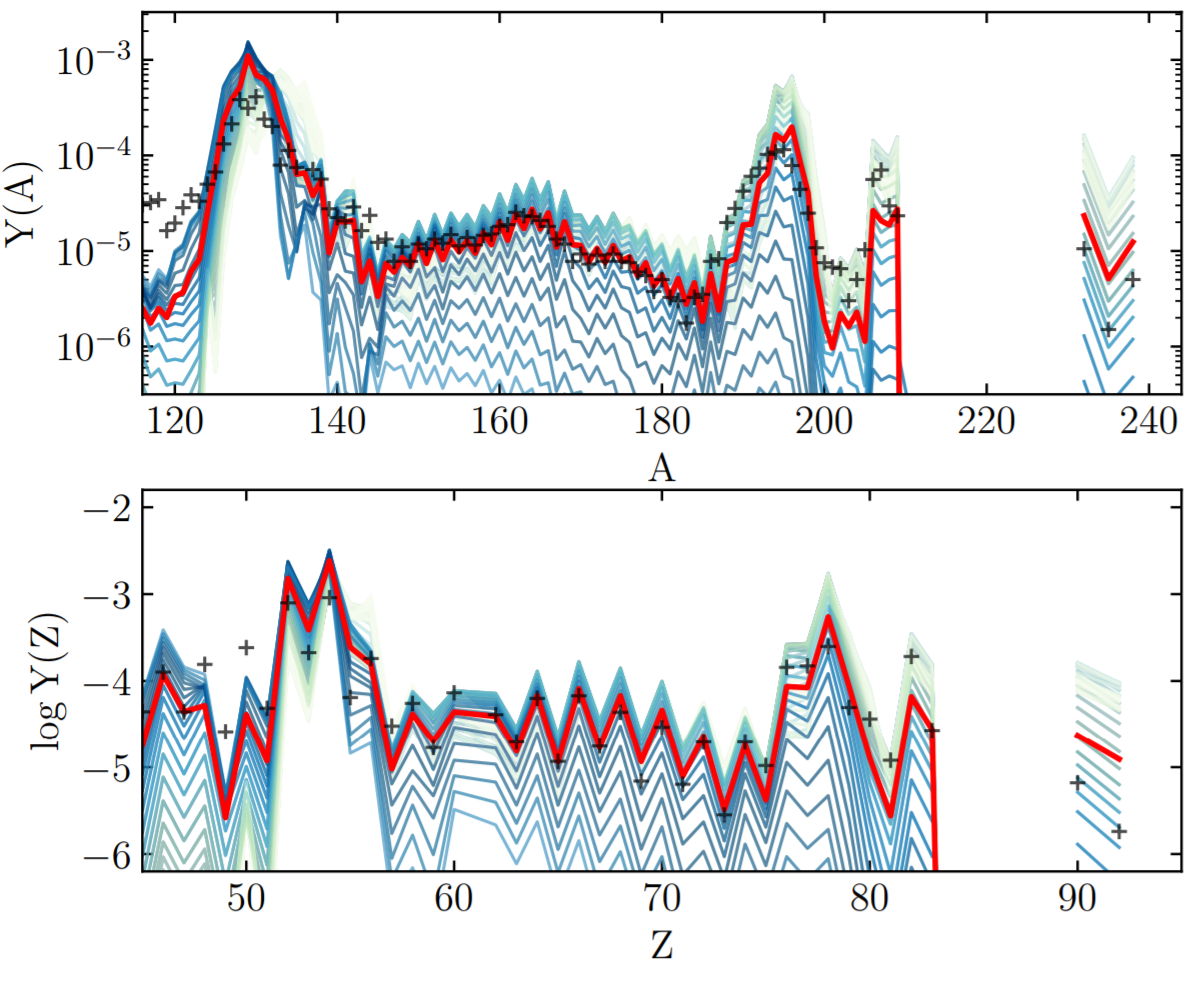In some simulations actinides seem to be overproduced versus lanthanides

A sufficient amount of dilution with ligher $r$-process material is required to match the solar isotopic residuals

∴ Fission theory has implications far beyond nucleosynthetic outcomes; e.g. for galactic chemical evolution, etc.

Holmbeck et al. ApJ 870 1 (2019) • Vassh et al. J. Phys. G (2019)

## Nuclear fission in a nutshell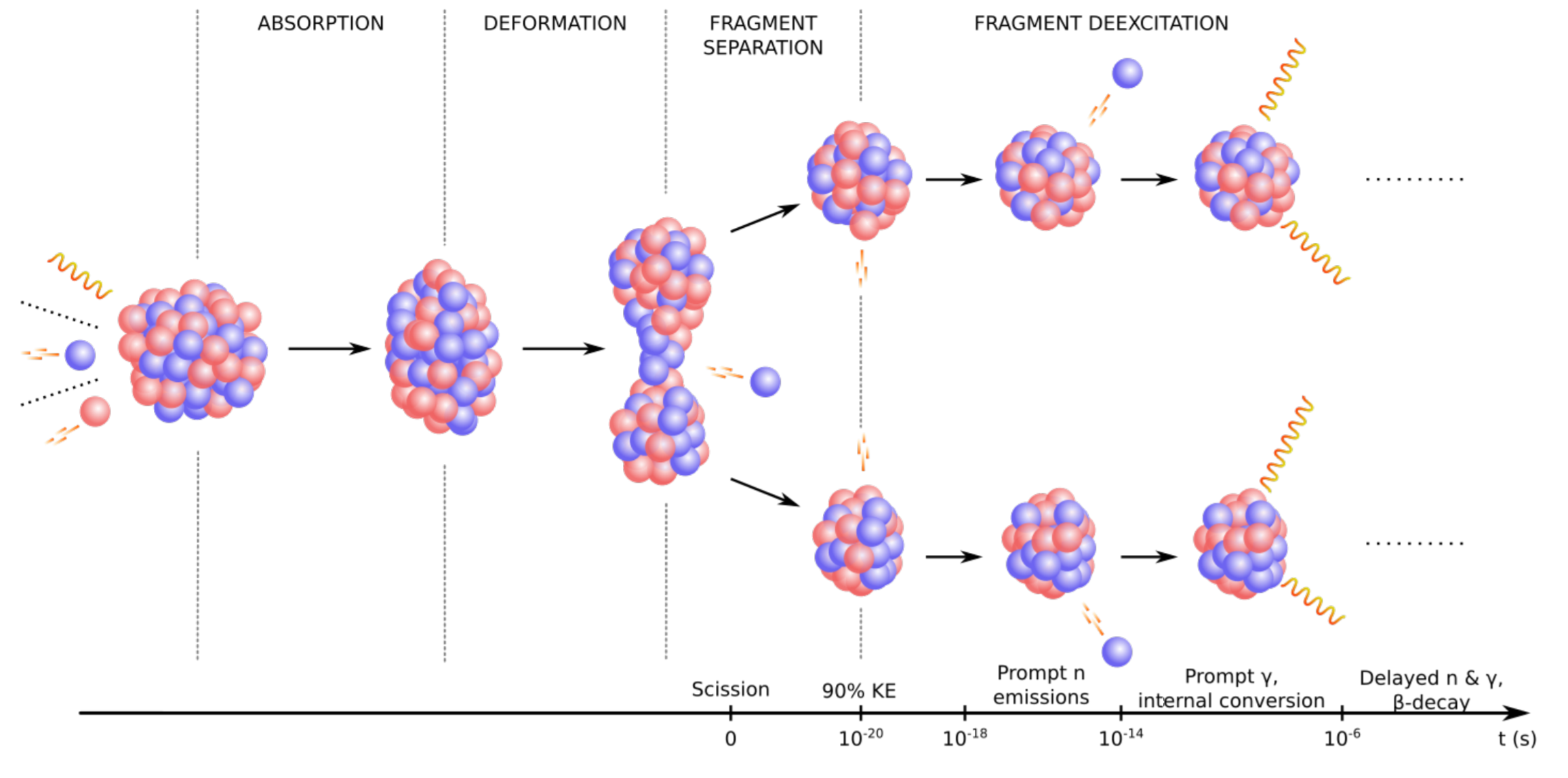The fission process:

A heavy nucleus splits into two lighter fragments

Subsequent particle emission and decays then occur

Many events gives rise to fission yield

Meitner & Frisch (1938) • Bohr & Wheeler (1939) • Figure from Verriere et al. in prep. (2019)

## Nuclear fission for the $r$-processInfluence on the $r$-process:

Fission rates and branching determine re-cycling (robustness)

Fragment yields place material at lower mass number; barriers determine hot spots

Large Q-value ⇒ impacts thermalization and therefore possibly observations

Responsible for what is left in the heavy mass region when nucleosynthesis is complete ⇒ "smoking gun"

Holmbeck et al. ApJ 870 1 (2019) • Vassh et al. J. Phys. G (2019) • Figure from Verriere et al. in prep. (2019)

## $\beta$ -delayed fission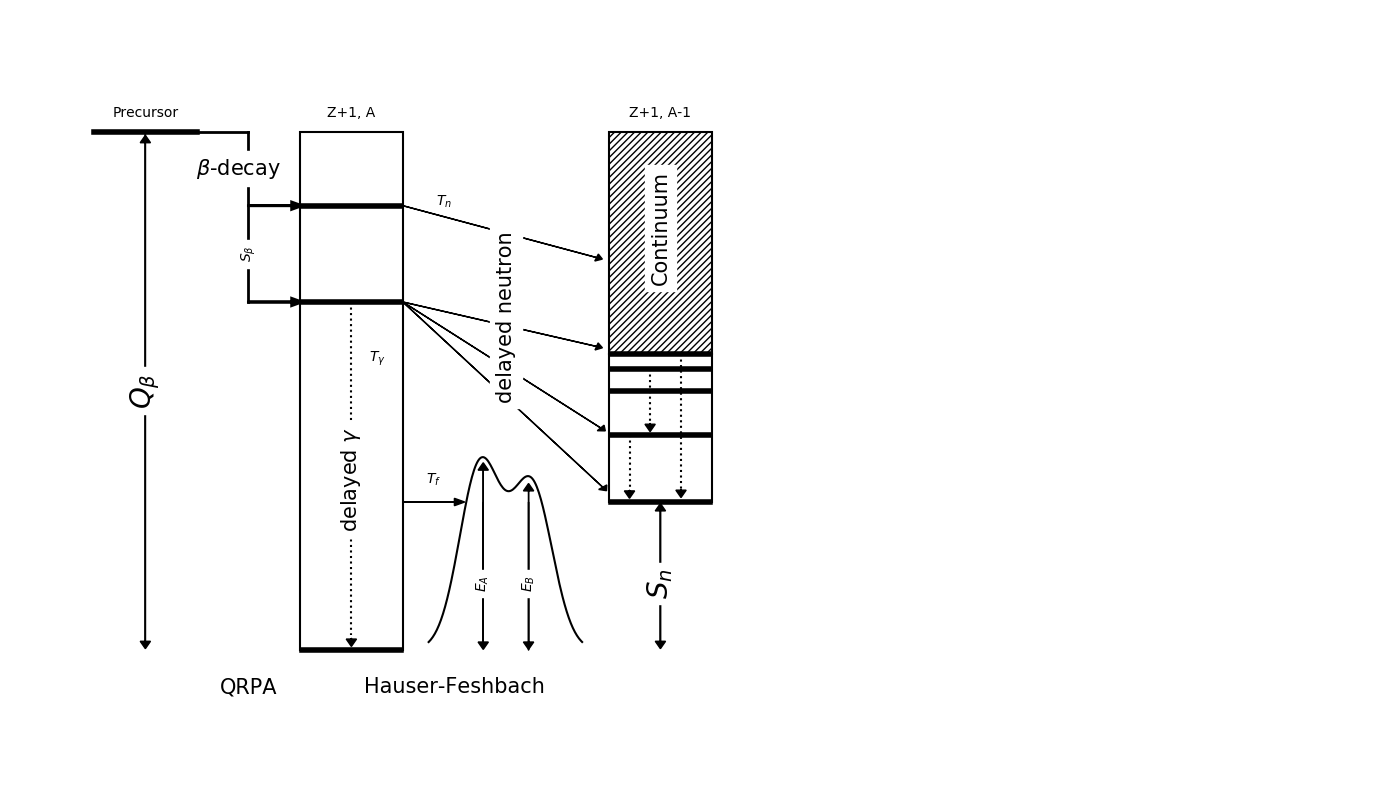We have recently extended our QRPA+HF model to describe $\beta$-delayed fission ($\beta$df)

Barrier heights from Möller et al. PRC 91 024310 (2015)

Assumes a Hill-Wheeler form for fission transmission

Mumpower et al. PRC 94 064317 (2016) • Spyrou et al. PRL (2016) • Möller et al. ADNDT 125 (2019)
Yokoyama et al. PRC (2019) • Mumpower et al. ApJ (2018)

## Multi-chance $\beta$ df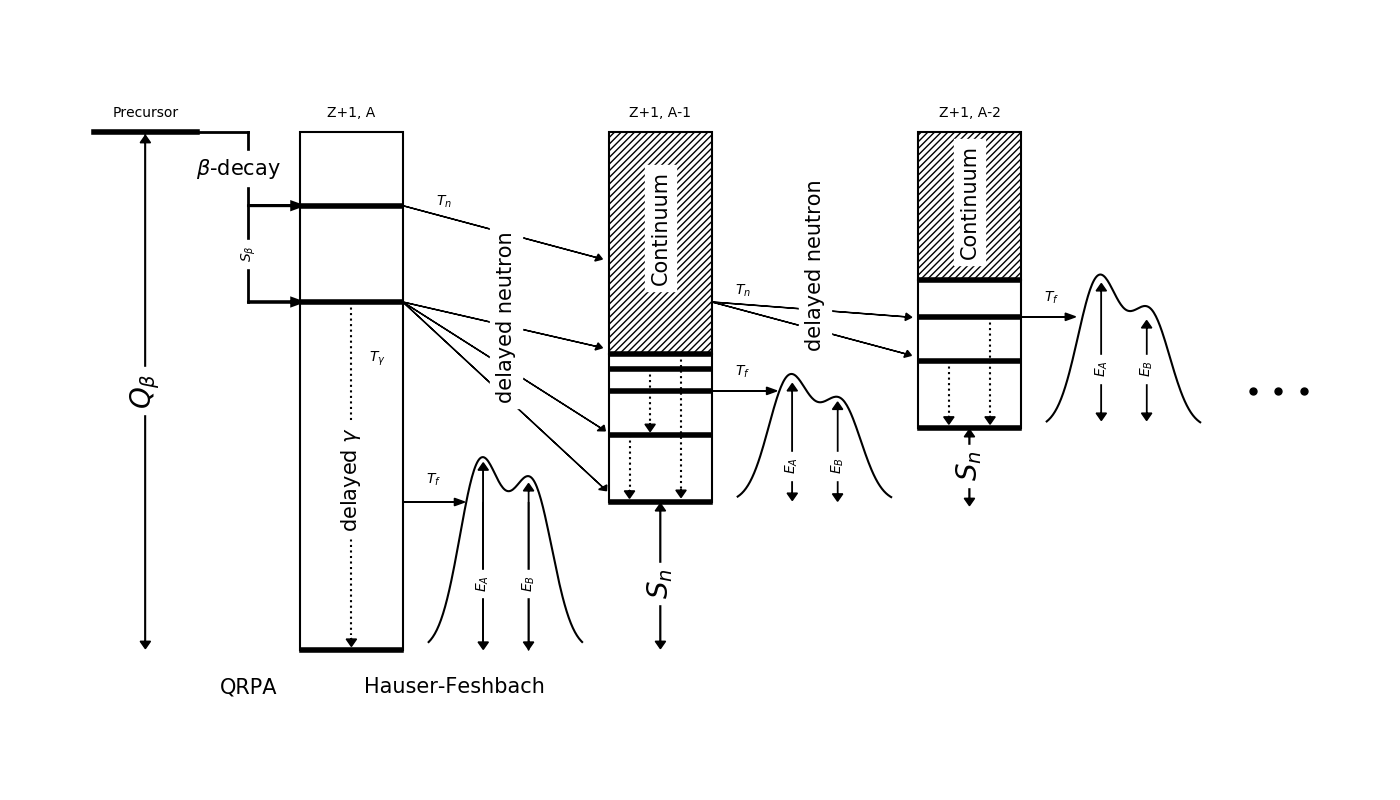Recall: Near the dripline $Q_{beta}$ ⇡ $S_{n}$ ⇣

Multi-chance $\beta$df: each daughter may fission

The yields in this decay mode are a convolution of many fission yields!

Mumpower et al. PRC 94 064317 (2016) • Spyrou et al. PRL (2016) • Möller et al. ADNDT 125 (2019)
Yokoyama et al. PRC (2019) • Mumpower et al. ApJ (2018)

## Cumulative $\beta$ df probability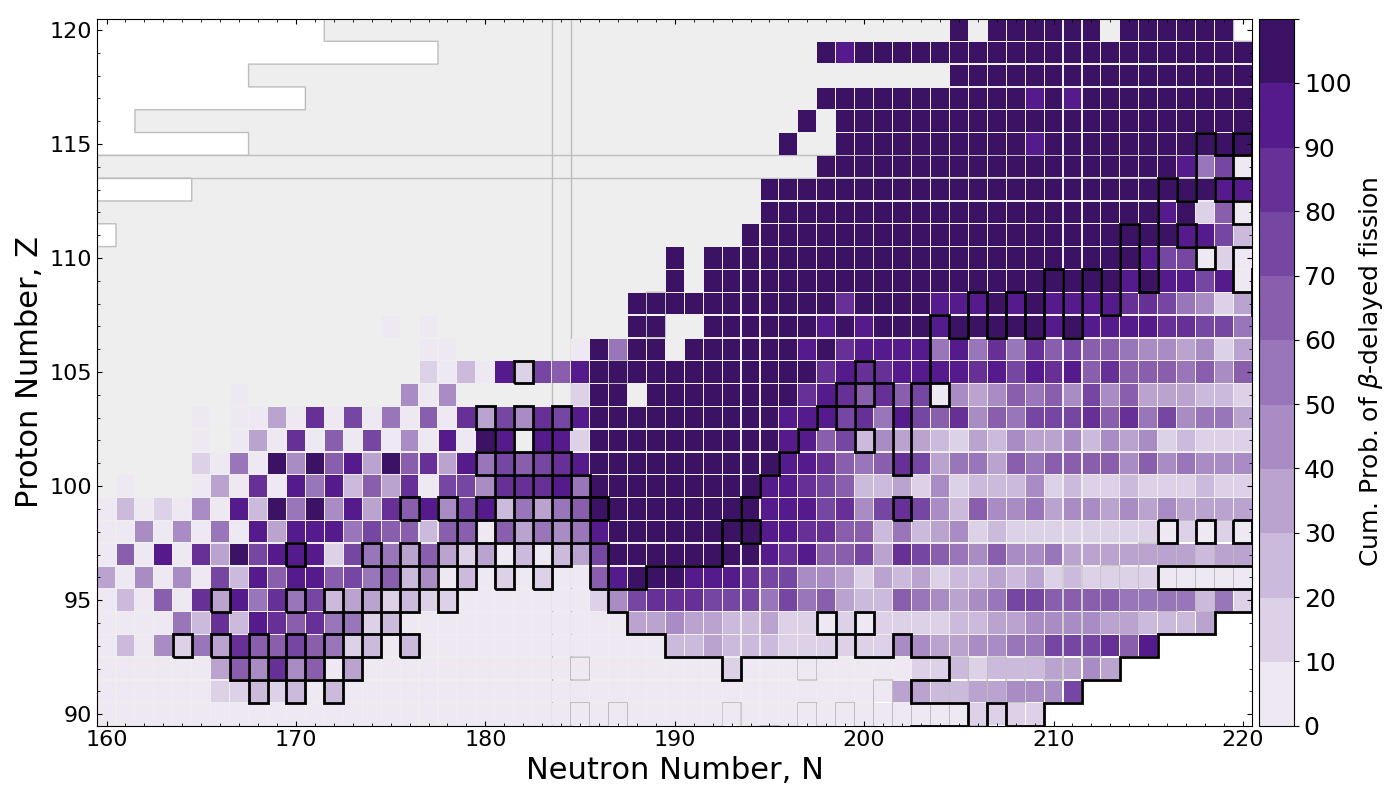$\beta$df occupies a large amount of real estate in the NZ-plane

Multi-chance $\beta$df outlined in black

Mumpower et al. ApJ 869 1 (2018)

## Application to the $r$-processPRISM network calculation of neutron star merger ejecta

$\beta$df alone may prevent the production of superheavy elements in nature

Thielemann et al. Zeitschrift fur Physik A Atoms and Nuclei 309 4 (1983) • Mumpower et al. ApJ 869 1 (2018)

## Impact on final abundances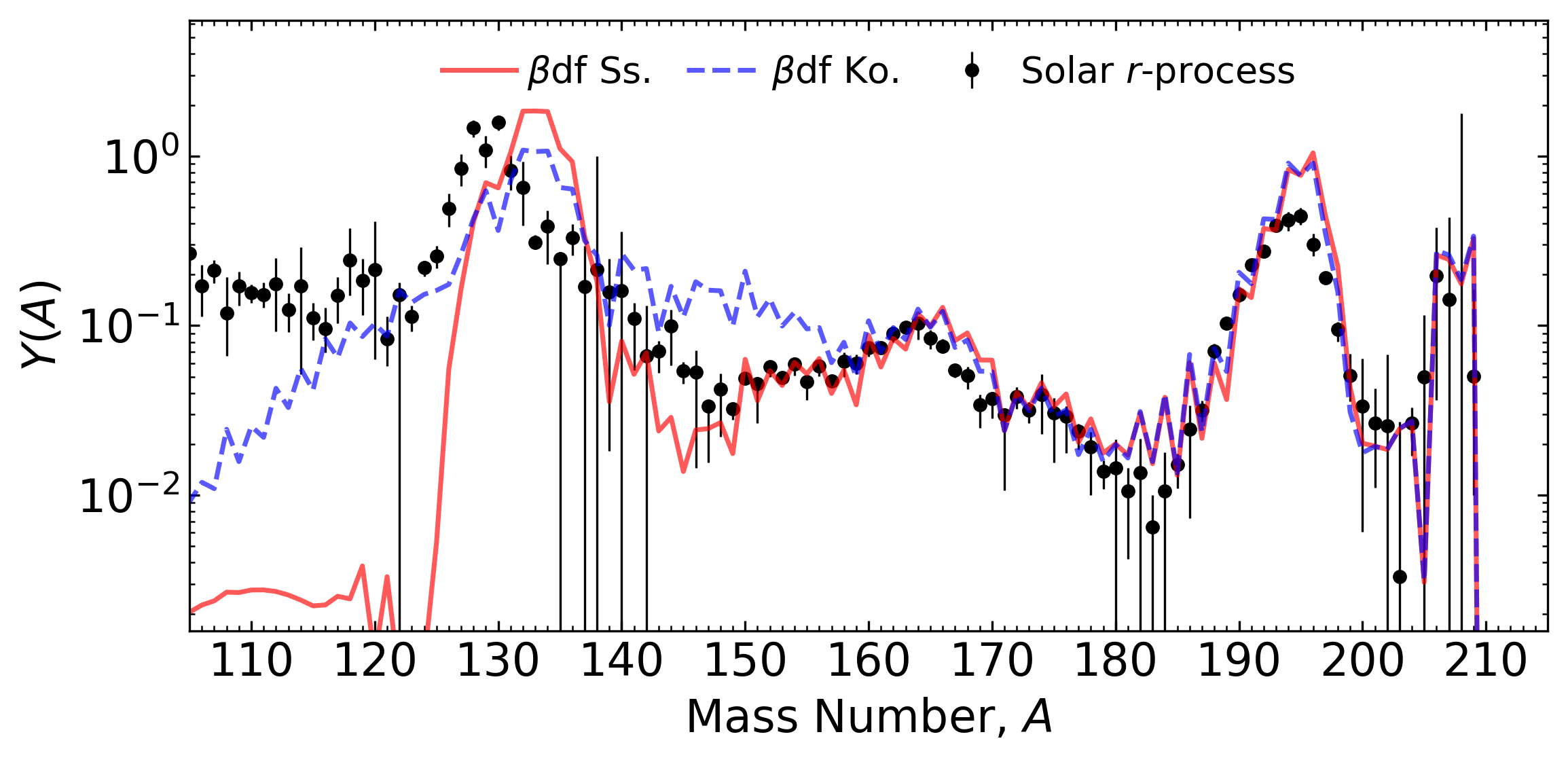Network calculation of tidal ejecta from a neutron star merger (FRDM2012)

$\beta$df can shape the final pattern near the $A=130$ peak

This is because of a relatively long fission timescale

Conclusion ⇒ we need a good description of fission yields to understand abundances near $A\sim130$.

Kodama & Takahashi (1975) • Shibagaki et al. ApJ (2016) • Mumpower et al. ApJ 869 1 (2018) • Vassh et al. J. Phys. G (2019)

## Fission hot spots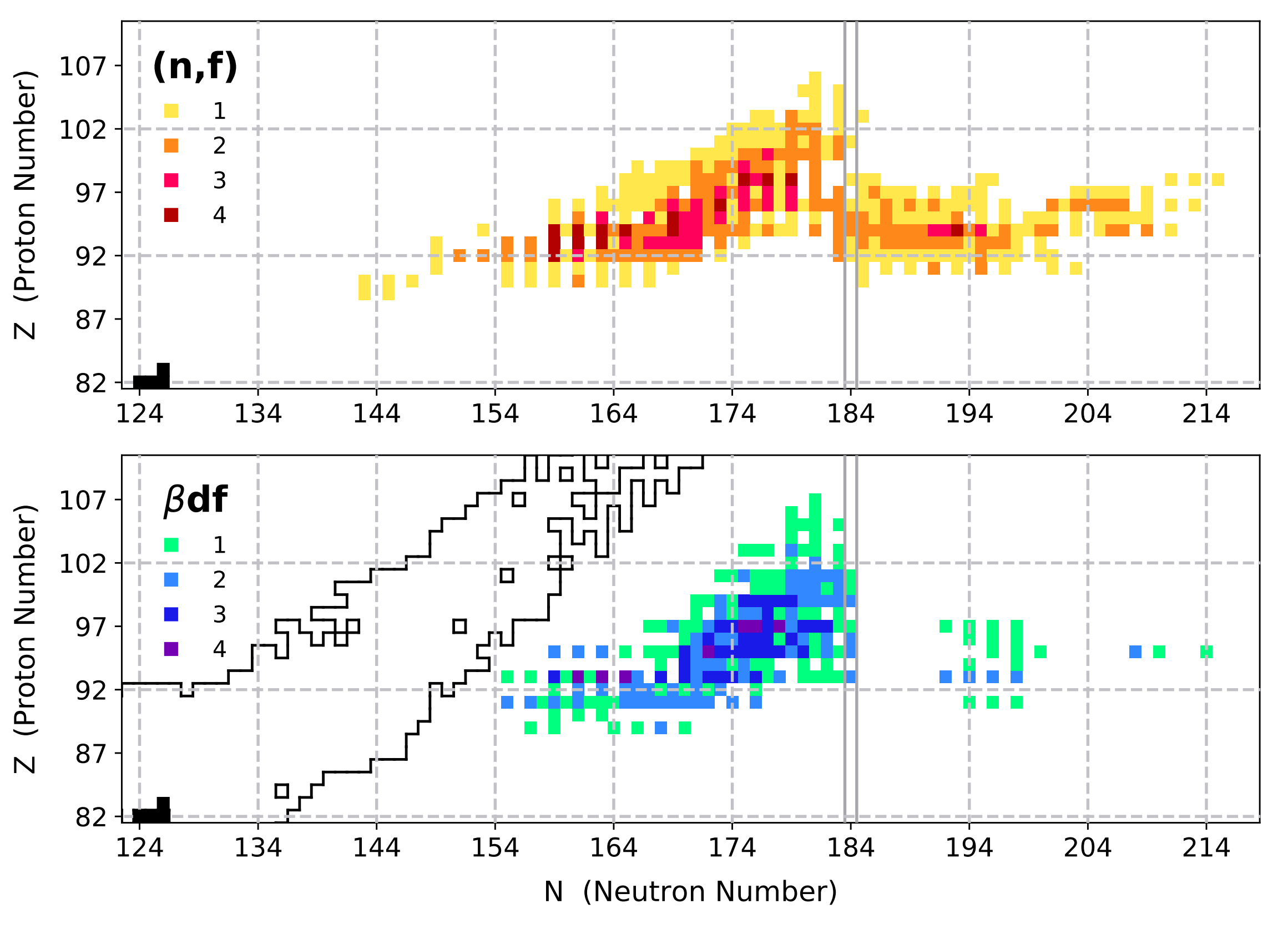We've taken a look at the region where fission seems to occur the most

With variations in both astrophysical conditions and nuclear models

Nuclei which influence the final abundances are colored for (n,f) and ($\beta$,f)

Vassh et al. J. Phys. G (2019)

## One example: $^{254}$Cf(Z=98)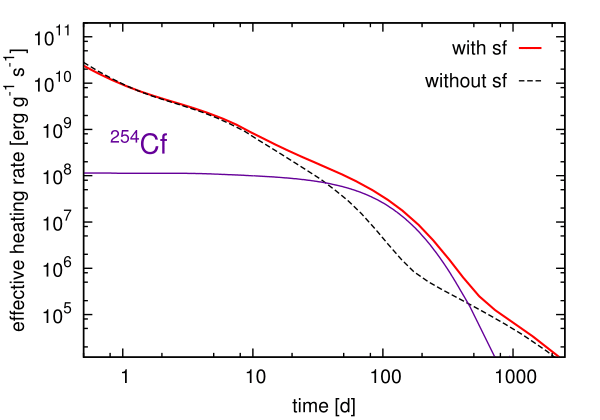Is there any possible precursor to show that actinide nucleosynthesis has occurred in an event?... Maybe!

The spontaneous fission of $^{254}$Cf can be a primary contributor to nuclear heating at late-time epochs

The $T_{1/2}\sim 60$ days; found from nuclear weapons testing

Baade et al. PASP (1956) • Conway et al. JOSA (1962) • Wanajo et al. ApJL (2014) • Y. Zhu et al. ApJL 863 2 (2018)
Vassh et al. J. Phys. G (2019)

## Production of $^{254}$Cf(Z=98)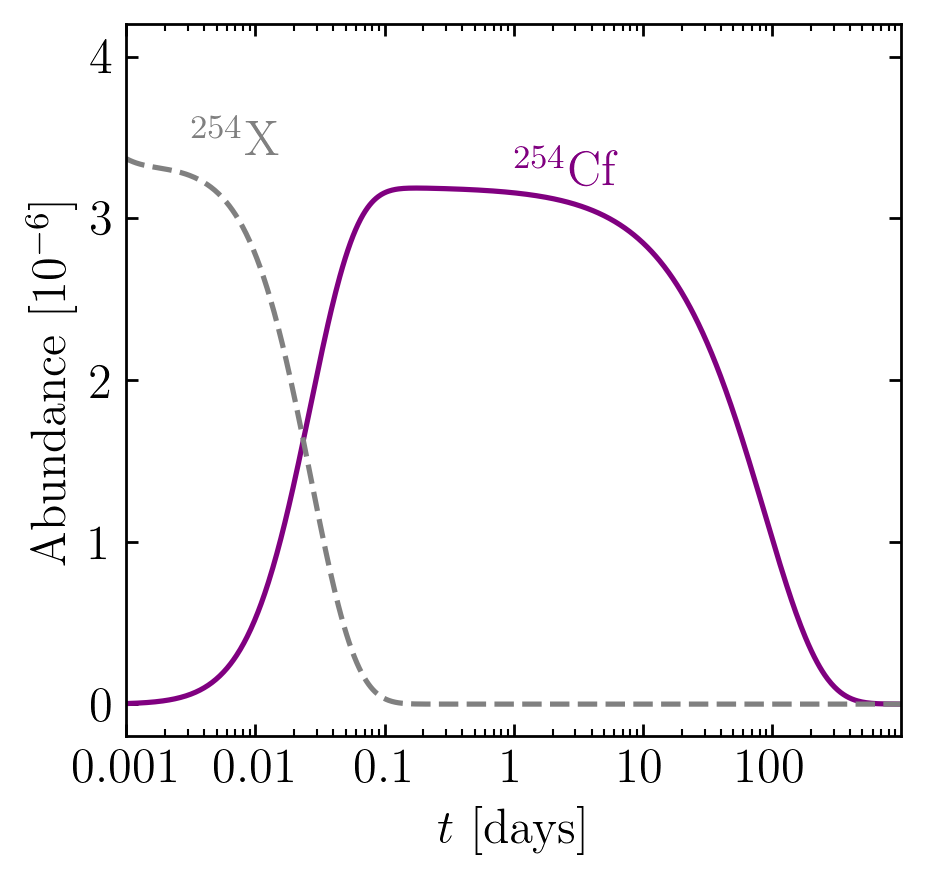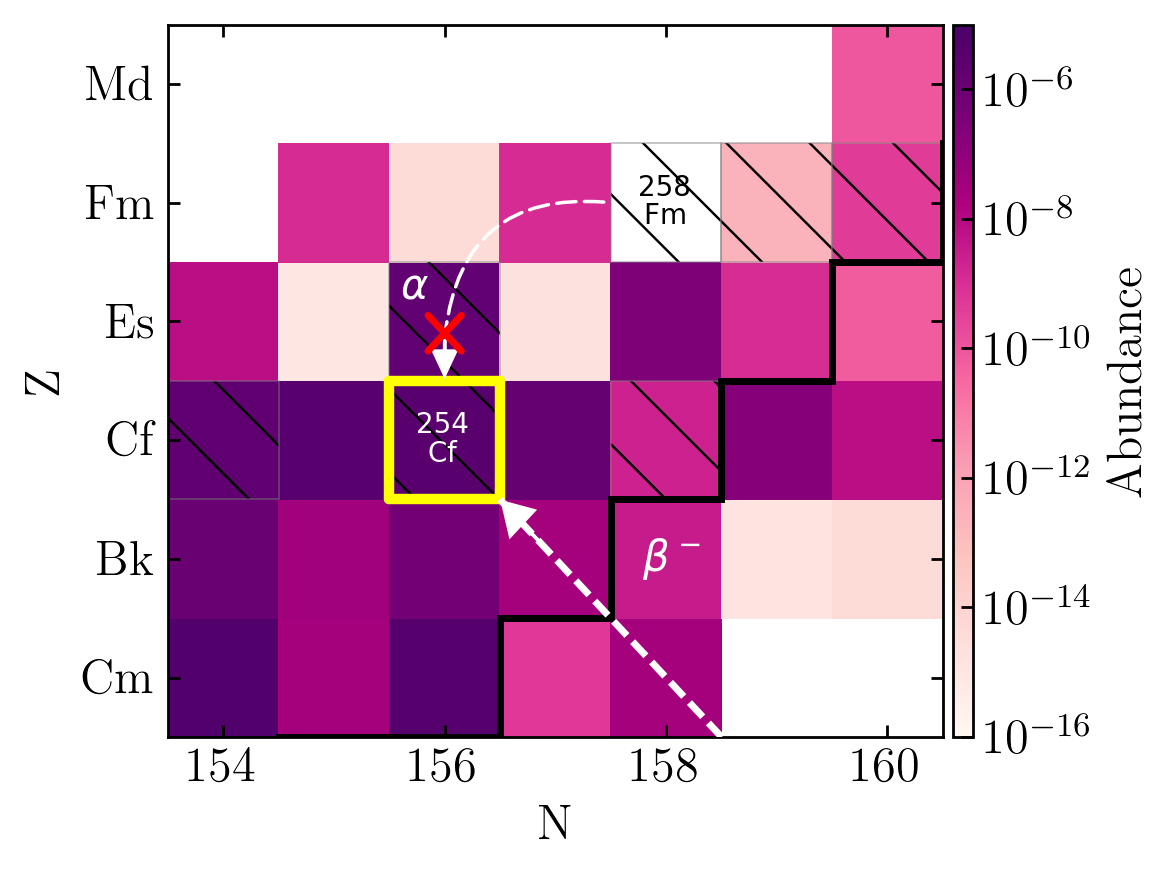Primary feeder seems to be from $\beta$-decay

Production of this nucleus been explored over a range of nuclear models; some high - some low

Remains to be seen if we can disentangle from other late-time heating sources (e.g. puslar or accreetion fallback)

Wanajo et al. (2014) • Y. Zhu et al. ApJL 863 2 (2018) • Vassh et al. J. Phys. G (2019) • Wollaeger et al. ApJ (2019) • Wu et al. PRL (2019)

## Production of $^{254}$Cf(Z=98)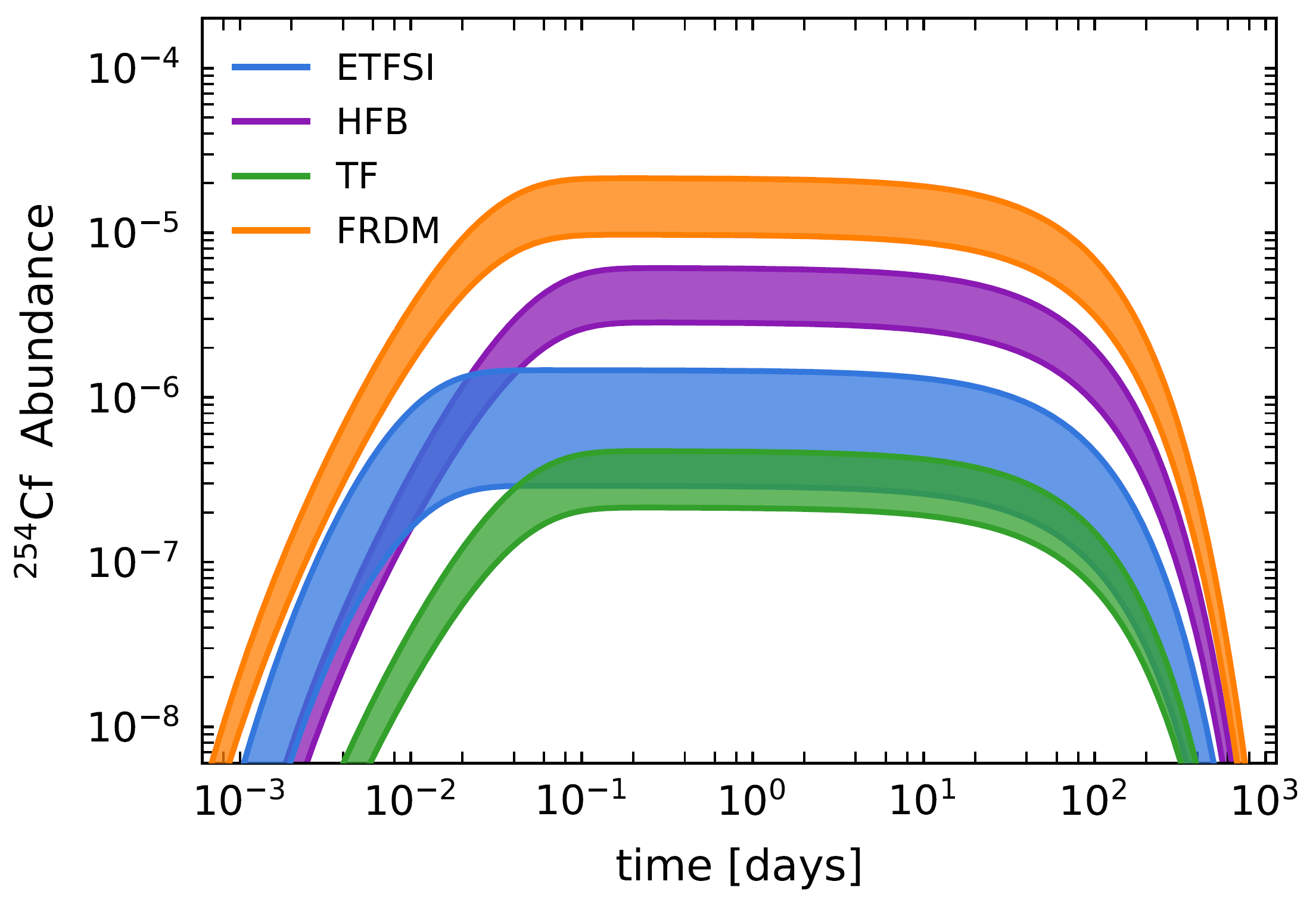We have also looked at the variation of production in this element with change in fission barriers

Vassh et al. J. Phys. G (2019)

## Observational Impact of Californium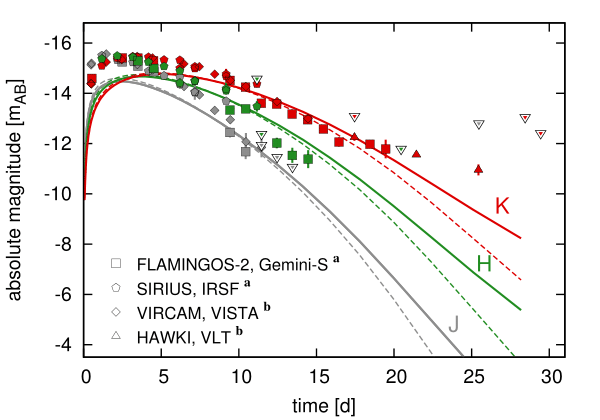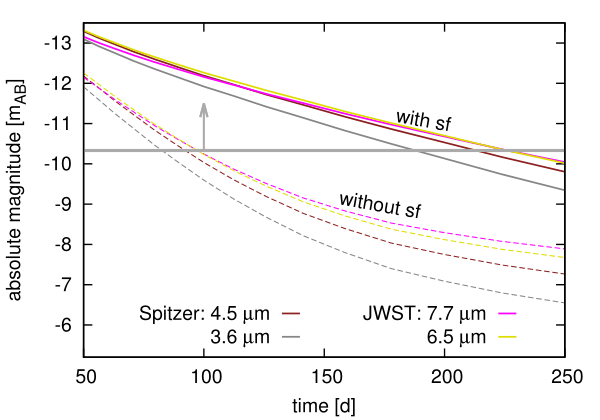Both near- and middle- IR are impacted by the presence of $^{254}$Cf

Late-time epoch brightness can be used as a proxy for actinide nucleosynthesis

Future JWST will be detectable out to 250 days with the presence of $^{254}$Cf

This also has implications for merger morphology...

Wanajo et al. (2014) • Y. Zhu et al. ApJL 863 2 (2018) • Vassh et al. J. Phys. G (2019) • Wollaeger et al. ApJ (2019) • Wu et al. PRL (2019)

## Merger $\gamma$-rays

Another possible (yet very difficult) option is to attempt to observe the spectra from transients / remnants

For the $r$-process we should search for signatures of actinides...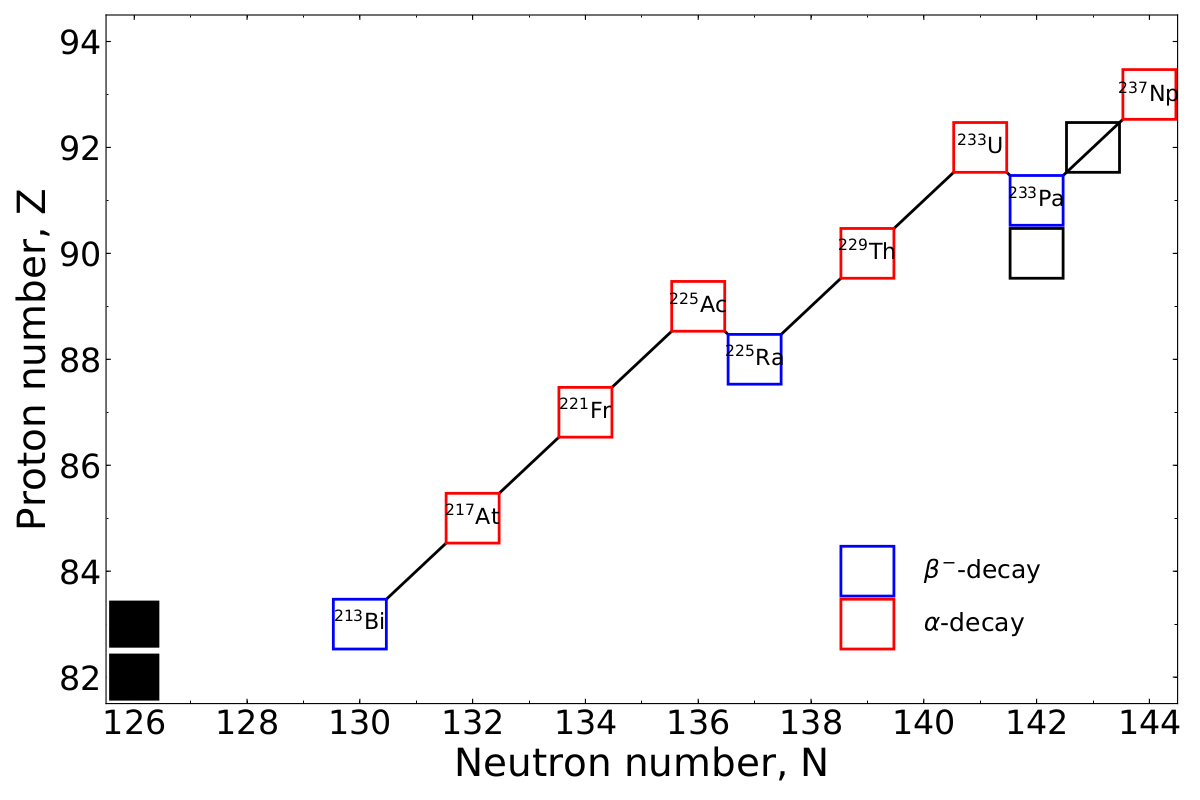This involves following potentially complex decay chains...

Wu et al. ApJ (2019) • Korobkin et al. accepted ApJ (2019)

## $\gamma$-ray spectrum at 10 kyr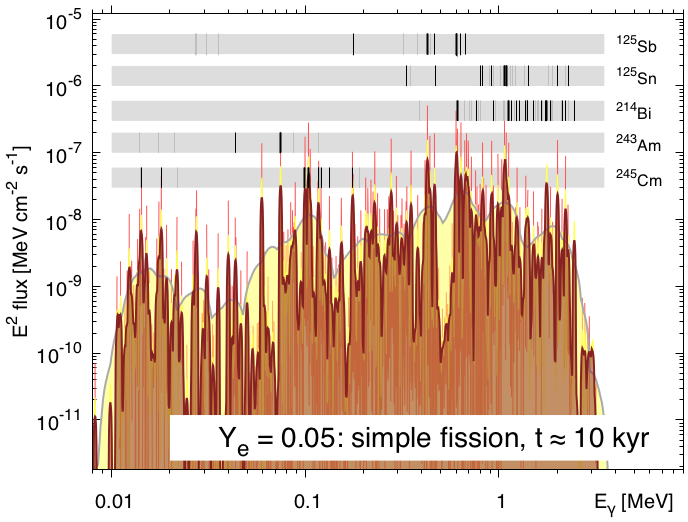Distinct signatures do arise; despite line broadening

This depends sensitively on observational timescale

Can we do this with future space missions?

Wu et al. ApJ (2019) • Korobkin et al. accepted ApJ (2019)

## Observing $\gamma$-rays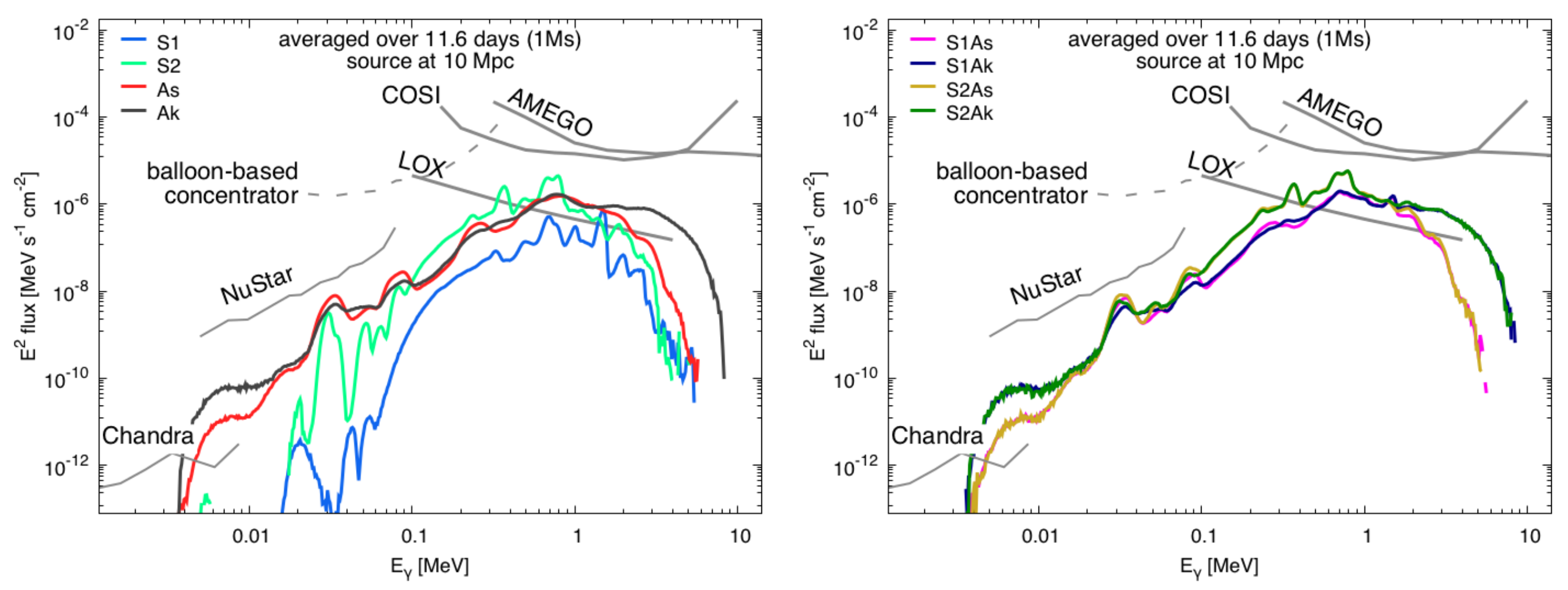Differing composition may be able to be ascertained, but the event has to be close (10 Mpc or less)

Possible candidates:

The Lunar Occultation Explorer (LOX)

Compton Spectrometer and Imager (COSI)

All-sky Medium Energy Gamma-ray Observatory (AMEGO) may all be promising

Korobkin et al. accepted ApJ (2019)

## Special thanks to

My collaborators

A. Aprahamian, J. Barnes, A. Burrows, J. Clark, B. Côté, J. Dolance, W. Even, C. Fontes, C. Fryer, E. Holmbeck, A. Hungerford, P. Jaffke, T. Kawano, O. Korobkin, J. Lippuner, G. C. McLaughlin, J. Miller, W. Misch, P. Möller, R. Orford, J. Randrup, G. Savard, T. Sprouse, R. Surman, N. Vassh, M. Verriere, R. Vogt, R. Wollaeger, Y. Zhu
& many more...

Student Postdoc CTA Staff FIRE PI

## Summary

The production of actinides in the $r$-process requires a deep understanding of nuclear physics

Recent insights include:

modeling of astrophysical environmentsMulti-messenger observations
Nuclear theory predictions

FRIB, etc. will help to constrain nuclear models, but the heaviest elements will remain relatively inaccessible

We therefore need to keep developing and studying theoretical models of nuclear physics

Nuclear modeling is absolutely crucial if we want to prove definitively that heavy elements such as the actinides were made in an event

Results / Data / Papers @ MatthewMumpower.com